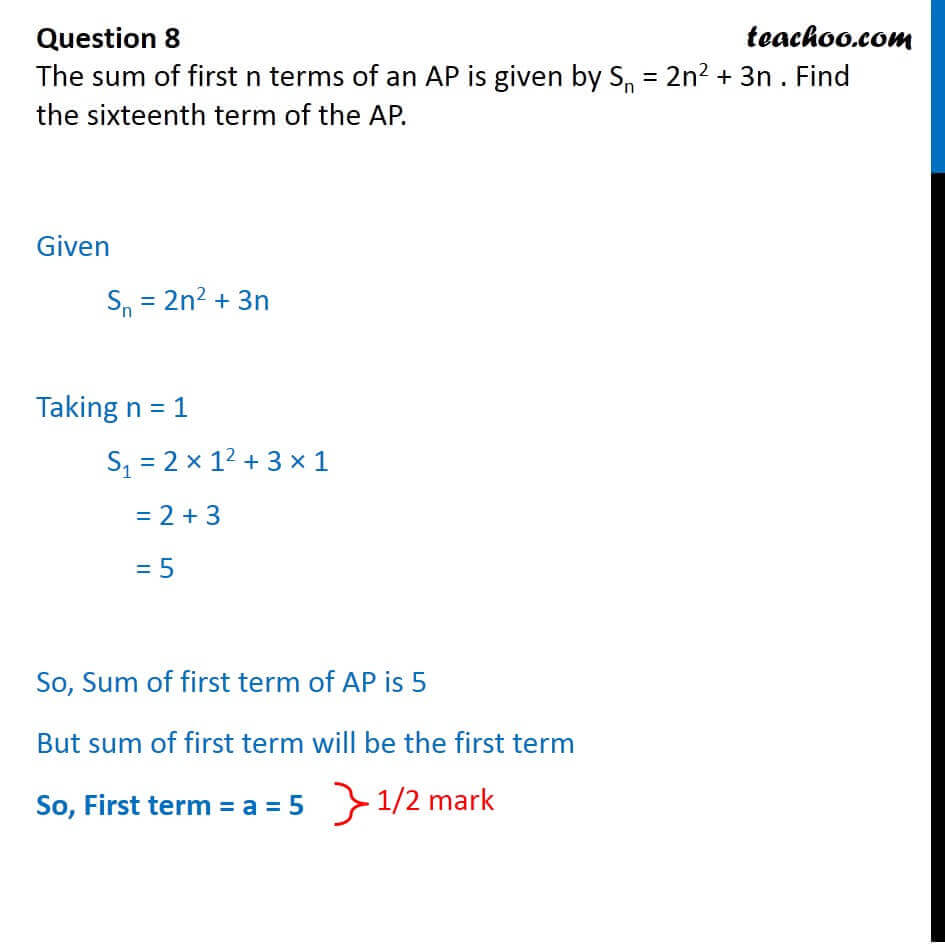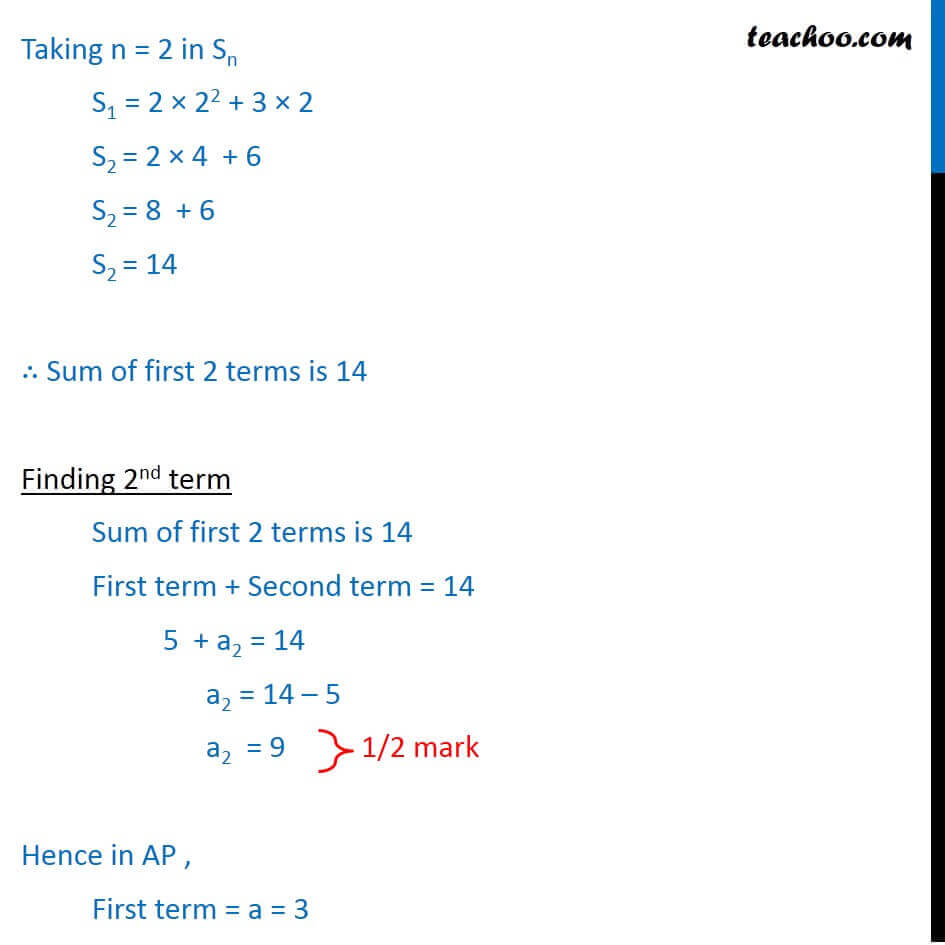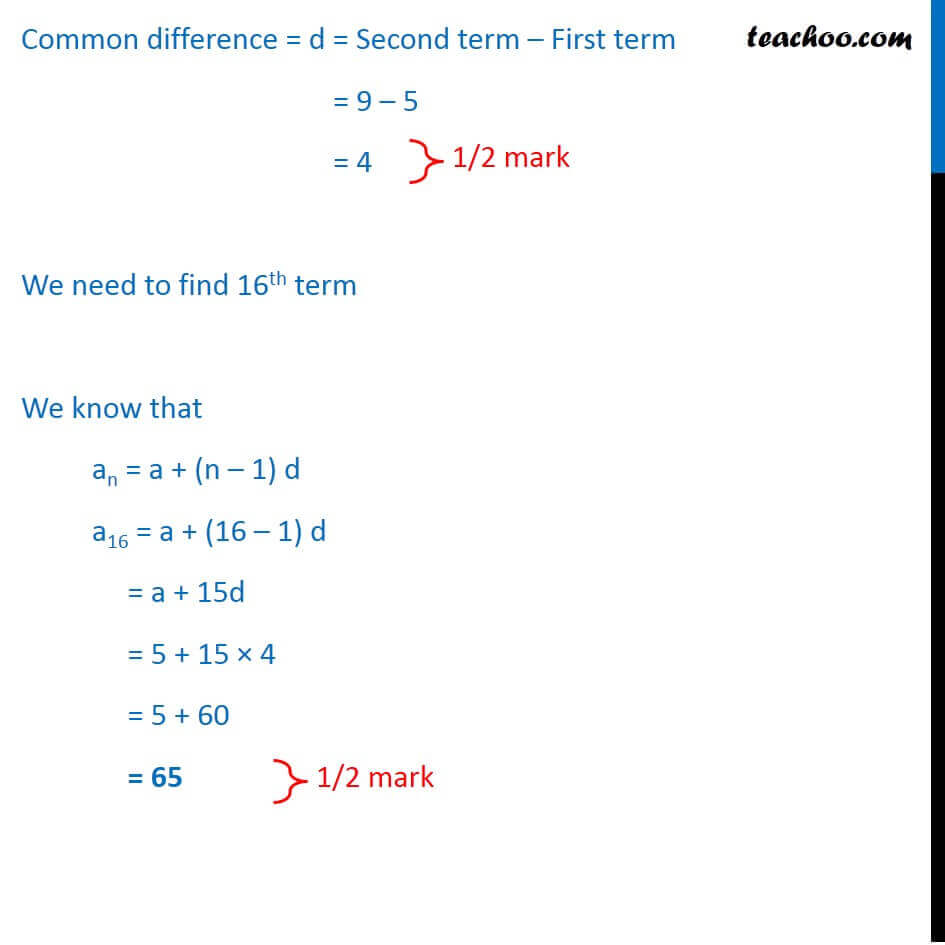CBSE Class 10 Sample Paper for 2018 Boards

Class 10
Solutions of Sample Papers for Class 10 Boards

### The sum of first n terms of an AP is given by S n = 2n 2 + 3n . Find the sixteenth term of the AP.

This is a question of CBSE Sample Paper - Class 10 - 2017/18.Learn in your speed, with individual attention - Teachoo Maths 1-on-1 Class

### Transcript

Question 8 The sum of first n terms of an AP is given by Sn = 2n2 + 3n . Find the sixteenth term of the AP. Given Sn = 2n2 + 3n Taking n = 1 S1 = 2 12 + 3 1 = 2 + 3 = 5 So, Sum of first term of AP is 5 But sum of first term will be the first term So, First term = a = 5 Taking n = 2 in Sn S1 = 2 22 + 3 2 S2 = 2 4 + 6 S2 = 8 + 6 S2 = 14 Sum of first 2 terms is 14 Finding 2nd term Sum of first 2 terms is 14 First term + Second term = 14 5 + a2 = 14 a2 = 14 5 a2 = 9 Hence in AP , First term = a = 3 Common difference = d = Second term First term = 9 5 = 4 We need to find 16th term We know that an = a + (n 1) d a16 = a + (16 1) d = a + 15d = 5 + 15 4 = 5 + 60 = 65